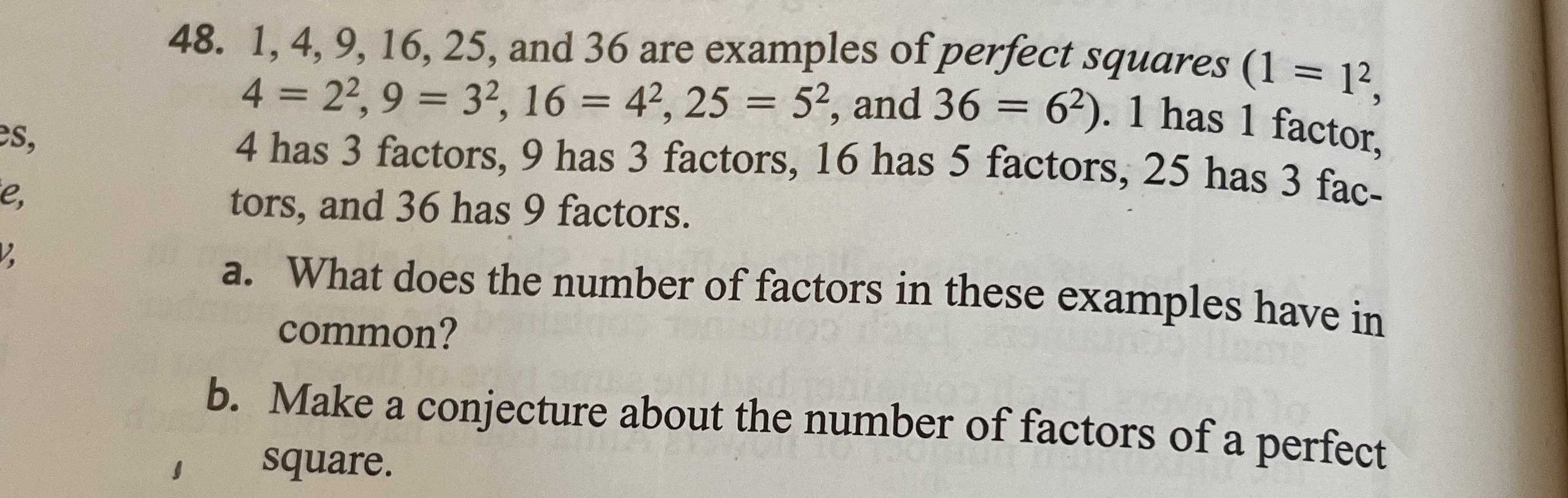### ¿Todavía tienes preguntas de matemáticas?

Pregunte a nuestros tutores expertos
Algebra
Pregunta48. $$1,4,9,16,25$$ , and $$36$$ are examples of perfect squares $$( 1 = 1 ^ { 2 }$$

$$4 = 2 ^ { 2 } , 9 = 3 ^ { 2 } , 16 = 4 ^ { 2 } , 25 = 5 ^ { 2 }$$ , and $$36 = 6 ^ { 2 } ) .1$$ has $$1$$ factor,

$$4$$ has $$3$$ factors, $$9$$ has $$3$$ factors, $$16$$ has $$5$$ factors, $$25$$ has $$3$$ fac- tors, and $$36$$ has $$9$$ factors. a. What does the number of factors in these examples have in common? b. Make a conjecture about the number of factors of a perfect square.